Marketing Product Price Promotion Place Price Price has

• Slides: 21Marketing. . . Product / Price / Promotion / PlacePrice: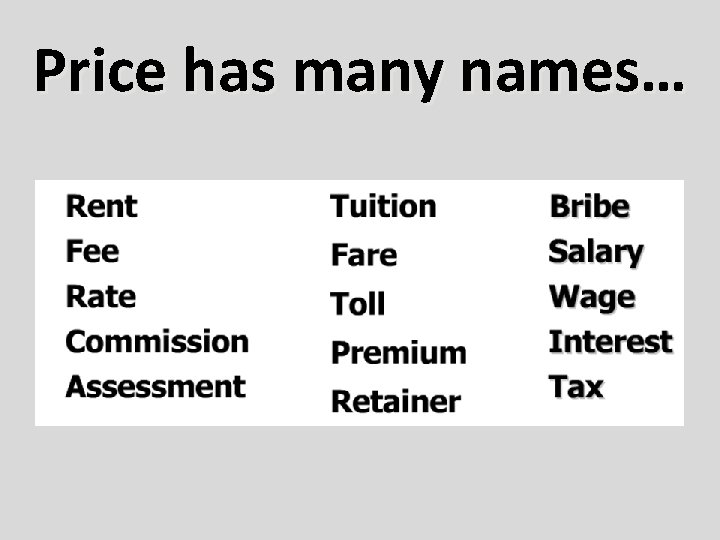Price has many names…DETERMINING THE PRICE Two key factors determine the price of an item: –the cost of doing business –the profit the company wants to make Simple formula: Price = Cost of Doing Business + Profit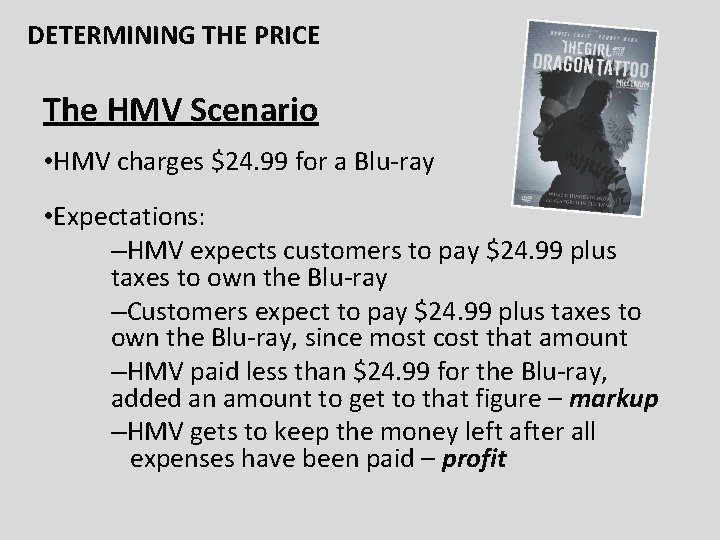DETERMINING THE PRICE The HMV Scenario • HMV charges \$24. 99 for a Blu-ray • Expectations: –HMV expects customers to pay \$24. 99 plus taxes to own the Blu-ray –Customers expect to pay \$24. 99 plus taxes to own the Blu-ray, since most cost that amount –HMV paid less than \$24. 99 for the Blu-ray, added an amount to get to that figure – markup –HMV gets to keep the money left after all expenses have been paid – profit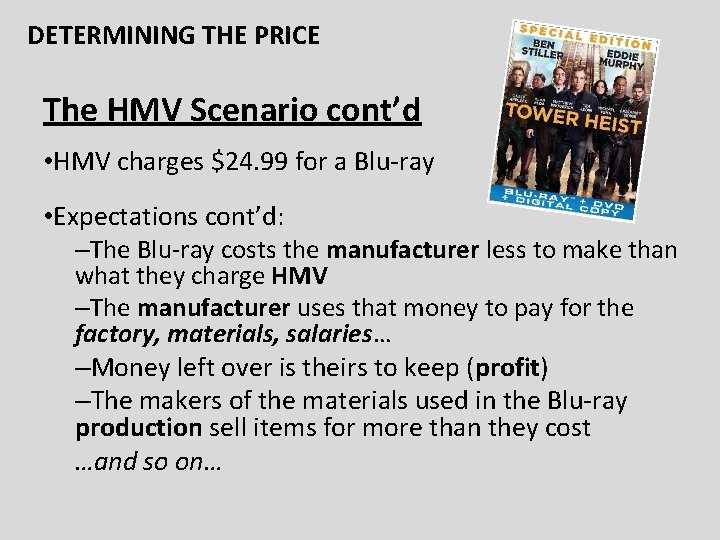DETERMINING THE PRICE The HMV Scenario cont’d • HMV charges \$24. 99 for a Blu-ray • Expectations cont’d: –The Blu-ray costs the manufacturer less to make than what they charge HMV –The manufacturer uses that money to pay for the factory, materials, salaries… –Money left over is theirs to keep (profit) –The makers of the materials used in the Blu-ray production sell items for more than they cost …and so on…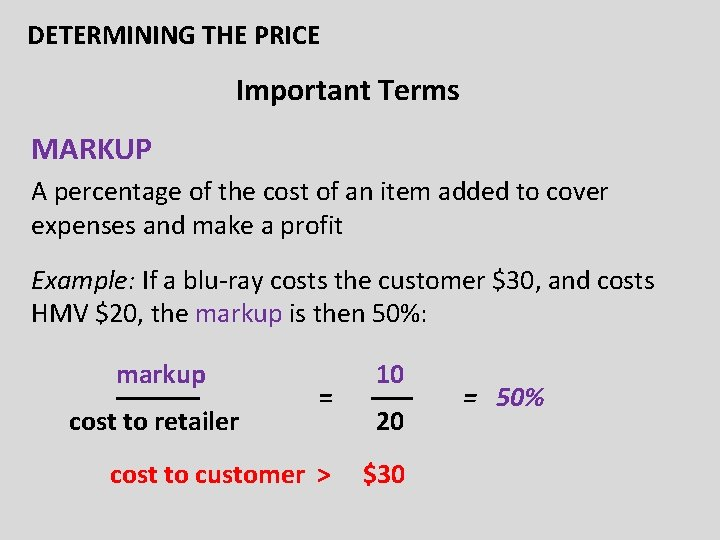DETERMINING THE PRICE Important Terms MARKUP A percentage of the cost of an item added to cover expenses and make a profit Example: If a blu-ray costs the customer \$30, and costs HMV \$20, the markup is then 50%: markup –––––– cost to retailer = 10 ––– 20 cost to customer > \$30 = 50%DETERMINING THE PRICE Important Terms cont’d… MARGIN The percentage of the price charged for the item which is not used to pay for the cost of the item Example: for a \$20 item, if customer pays \$30 there is a \$10 markup and the margin would be: markup ––––– selling price = \$10 ––– = 33. 3% \$30DETERMINING THE PRICE Important Terms cont’d GROSS PROFIT Money left over after all “variable costs” have been paid. business gross profit = markup expenses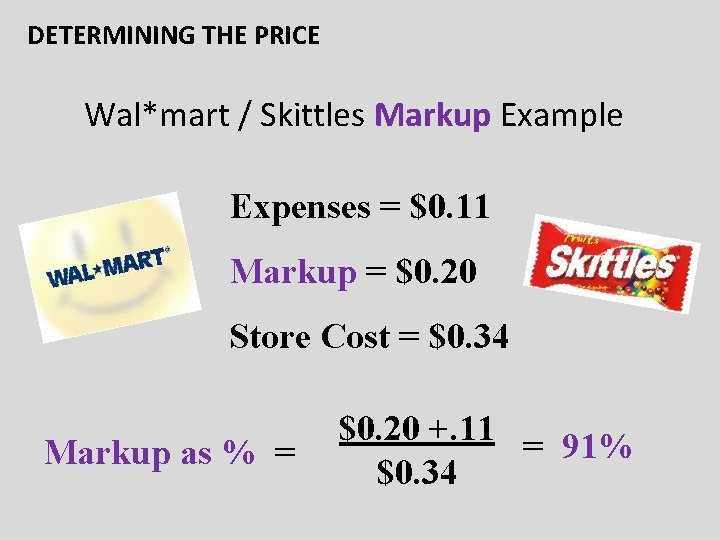DETERMINING THE PRICE Wal*mart / Skittles Markup Example Expenses = \$0. 11 Markup = \$0. 20 Store Cost = \$0. 34 Markup as % = \$0. 20 +. 11 = 91% \$0. 34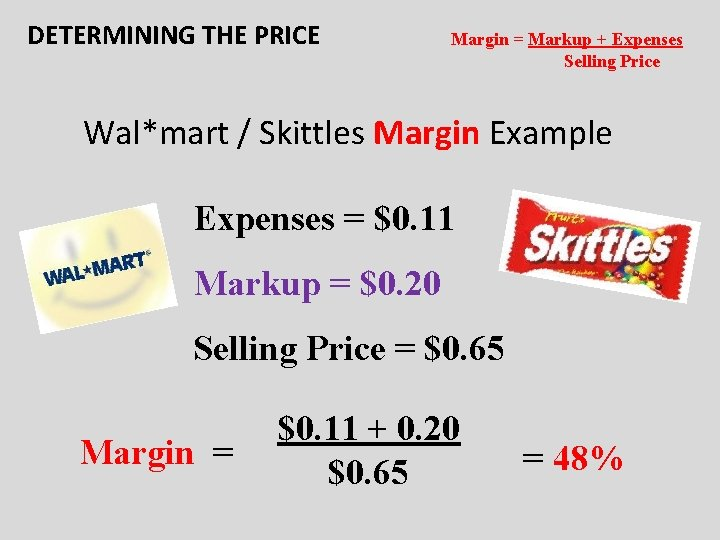DETERMINING THE PRICE Margin = Markup + Expenses Selling Price Wal*mart / Skittles Margin Example Expenses = \$0. 11 Markup = \$0. 20 Selling Price = \$0. 65 Margin = \$0. 11 + 0. 20 \$0. 65 = 48%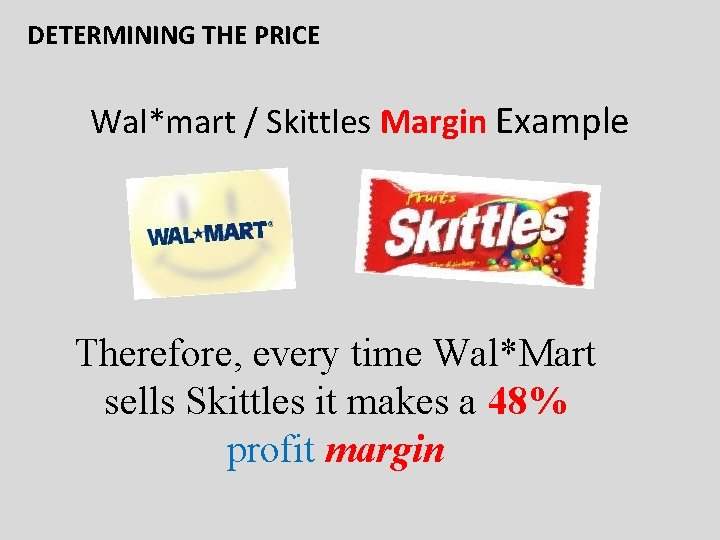DETERMINING THE PRICE Wal*mart / Skittles Margin Example Therefore, every time Wal*Mart sells Skittles it makes a 48% profit margin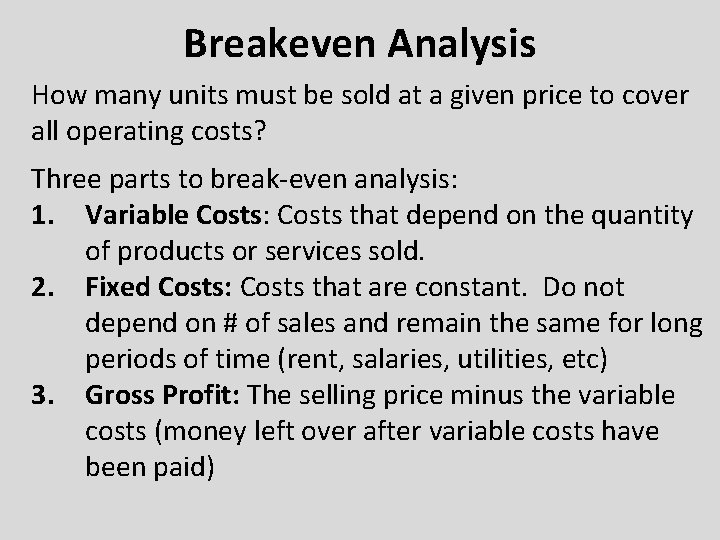Breakeven Analysis How many units must be sold at a given price to cover all operating costs? Three parts to break-even analysis: 1. Variable Costs: Costs that depend on the quantity of products or services sold. 2. Fixed Costs: Costs that are constant. Do not depend on # of sales and remain the same for long periods of time (rent, salaries, utilities, etc) 3. Gross Profit: The selling price minus the variable costs (money left over after variable costs have been paid)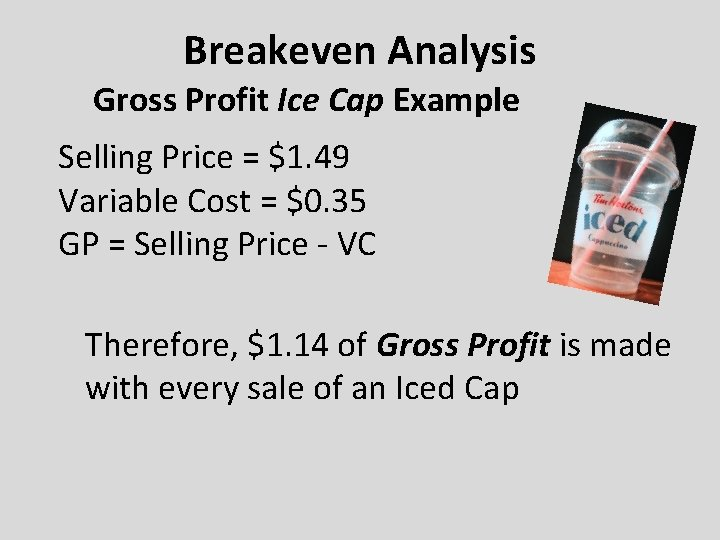Breakeven Analysis Gross Profit Ice Cap Example Selling Price = \$1. 49 Variable Cost = \$0. 35 GP = Selling Price - VC Therefore, \$1. 14 of Gross Profit is made with every sale of an Iced Cap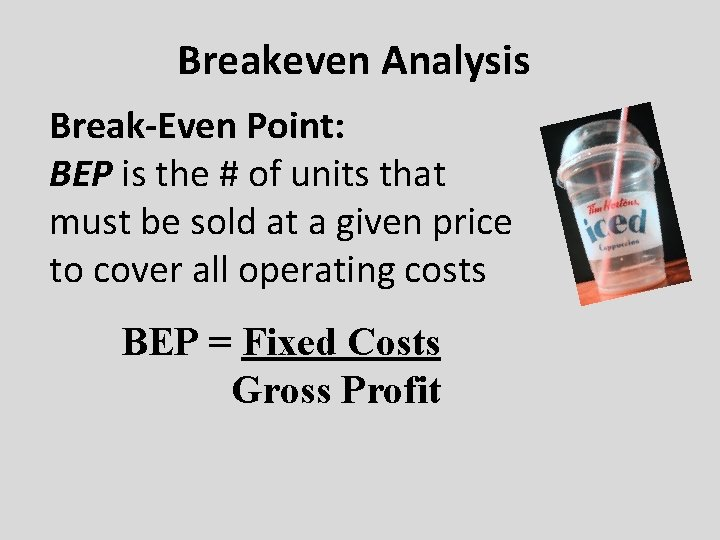Breakeven Analysis Break-Even Point: BEP is the # of units that must be sold at a given price to cover all operating costs BEP = Fixed Costs Gross ProfitBreakeven Analysis The BEP for just Ice Caps is hard to calculate because Tim Horton’s sells many other items ( Bagels, donuts, coffee, etc), however, lets say a typical Tim’s has a fixed cost of \$57 on Ice Cap sales per day: \$57 (Fixed Costs) = 50 (BEP) \$1. 14 (Gross Profit) Tim’s must sell 50 Ice Caps per day to reach the BEP.Breakeven Analysis Subway Pricing and BEP Example Subway has the following costs for a “Footlong” assorted sub it sells: Bread = \$0. 27 Meat = \$1. 08 Toppings = \$0. 20 Expenses = \$1. 05 (includes all other VC ) Subway wants to make \$2. 40 per sub. What should the price be? 0 0. \$5 ! … h DuBreakeven Analysis Subway Pricing and BEP Example What is subway’s cost of a footlong? (. 27+1. 08+. 20)= \$1. 55 What is the margin? (1. 05+2. 40)/5= 69% [ Margin = Markup + Expenses / Selling Price ] Total Variable costs? 1. 55+1. 05=\$2. 60 What is the markup? \$3. 45 As a percentage: (2. 40+1. 05)/1. 55= 222% The Numbers: Base Costs: Bread = \$0. 27 Meat = \$1. 08 Toppings = \$0. 20 Expenses = \$1. 05 (includes all VC) Subway MARKUP = \$2. 40 Cost to customer = \$5. 00 [ cost of an item added to cover expenses and make a profit / base cost of item ] What is the gross profit? 5 – 2. 60 = 2. 40Breakeven Analysis Subway Pricing and BEP Example So what is the BEP? Assume Subway pays the following monthly Fixed Costs: Wages \$10, 400 Rent \$1, 900 Hydro \$650 FC = \$12, 950 BEP = \$ 12, 950 / \$2. 40 = 5, 395 subs Therefore, Subway needs to sell 5, 395 subs per month just to break even! If a subway is open 30 days a month that would require a typical subway to sell 180 subs a day (or 15 an hour) to reach the BEP.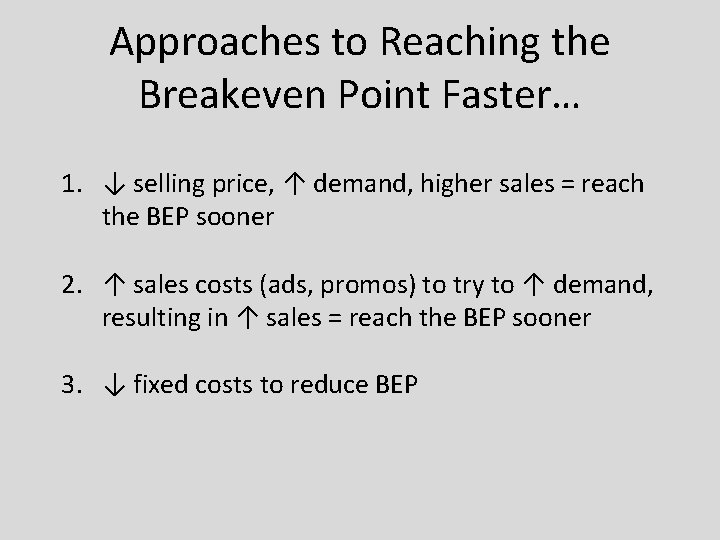Approaches to Reaching the Breakeven Point Faster… 1. ↓ selling price, ↑ demand, higher sales = reach the BEP sooner 2. ↑ sales costs (ads, promos) to try to ↑ demand, resulting in ↑ sales = reach the BEP sooner 3. ↓ fixed costs to reduce BEP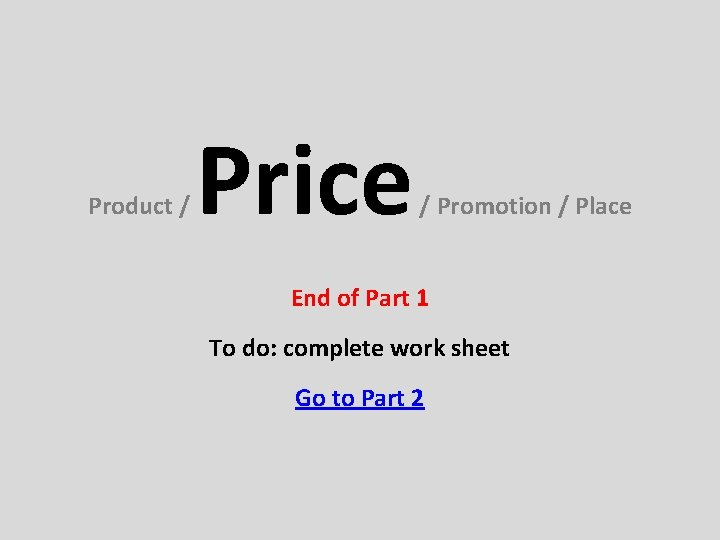Product / Price / Promotion / Place End of Part 1 To do: complete work sheet Go to Part 2